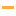Math Games

Play the best free online Math Games! Check out the Top 100 Math games from PrimaryGames.

#1Math Lines
#2Fraction MatcherMatch shapes and numbers to earn stars in this fractions game. Challenge yourself on any level you like. Try to collect lots of stars!

#3Math Mosaics: ChristmasIt's color by number! To determine the color of each space solve each addition problem, then use the code at the side of the page. The final picture will be revealed as each square is filled.

#4Animals Dot to Dot Puzzle
#5Pizza Party FractionsGet ready for a Pizza Party! Click on the fraction that shows the amount of pizza that is left.

#6What Time Is It?Look at the analog clock and tell the time. Then pick the digital clock that shows the same time.

#7Times Tables DiplomaPractice multiplication and earn your Times Table Diploma! Learn all the multiplication facts and become a multiplication expert.

#8Math Mosaics: WinterIt's color by number! To determine the color of each space solve each addition problem, then use the code at the side of the page. The final picture will be revealed as each square is filled.

#9Turkey TwosCount by 2s to fill in the missing numbers. Drag the correct number to fill in the missing spots.

#10Autumn Leaves MemoryFind the matching cards. Count up the leaves shown and find the card that shows the same number.

#11Turkey Fives
#12Math Maze: CountingPractice counting in the Math Maze. Start in the upper left corner and move through the maze by clicking on the number that comes next.

#13Santa CountIt
#14Turkey Tens
#15Math UpRace your way to the top by answer math questions correctly. Solve the addition, subtraction or multiplication problem then move the ball to the correct answer.

#16Fishy Count
#17Math BuzzUse your math skills to protect the queen and collect pollen throughout 5 unique worlds.

#18Pattern ManiaLook at the pattern and decide what comes next. Click on a picture to complete the pattern.

#19Math Mosaics: Valentine's DayIt's color by number! To determine the color of each space solve each addition problem, then use the code at the side of the page. The final picture will be revealed as each square is filled.

#20Math Tiles: Groundhog Day Addition and SubtractionPractice addition and subtraction skills in this fun puzzle game. Select from adding, subtracting or both and choose between a range of 10, 20 or 100.